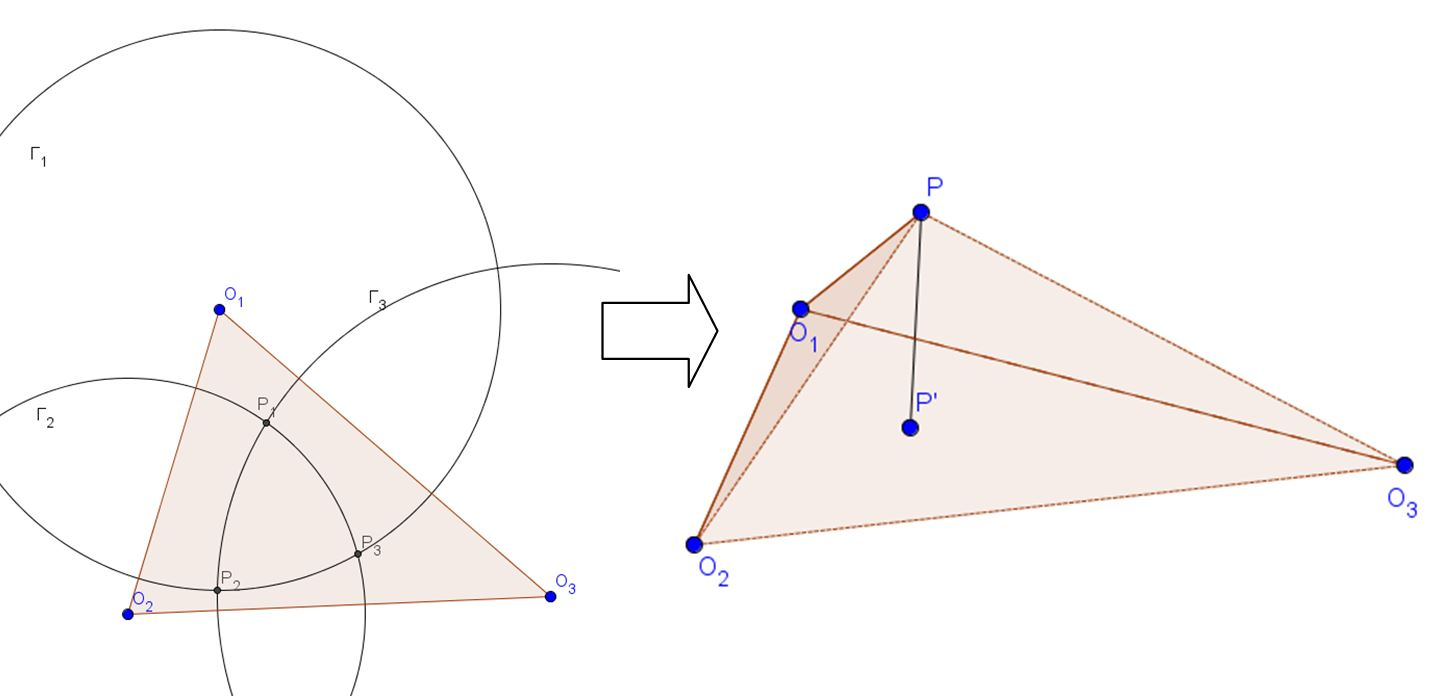# Three Dimensional Proof: Radical Axis Theorem

The Radical Axis Theorem states the following:

Given three distinct circles $\Gamma_1, \Gamma_2, \Gamma_3$, the radical axes of the three circles taken pairwise concur at a point, called the radical center.

The vanilla method of proving this statement is not hard:

Suppose $\Gamma_1$ and $\Gamma_2$ has radical axis $\ell_3$, and $\Gamma_2$ and $\Gamma_3$ has radical axis $\ell_1$. Let $P=\ell_1\cap \ell_3$. Then by the definition of radical axis, $\text{pow}(P, \Gamma_1)=\text{pow}(P, \Gamma_2)$ because $P\in\ell_3$ and $\text{pow}(P, \Gamma_2)=\text{pow}(P, \Gamma_3)$ because $P\in \ell_1$. Naturally, this means $\text{pow}(P, \Gamma_1)=\text{pow}(P, \Gamma_3)$ so $P\in\ell_2$, and the proof is finished.

Amazingly, there is also a very nice alternate way to prove this statement by extending the diagram to three dimensions. This proof will only work if the three circles pairwise intersect, but I believe it's possible to extend the proof to any three circles using complex points. But let's focus on real situations for now.Let the center of circle $\Gamma_i$ be $O_i$ for $1\le i\le 3$. In addition, let the intersection of circles $\Gamma_1$ and $\Gamma_2$ that is closer to $O_3$ be $P_3$, and define $P_1, P_2$ similarly.

Note that by definition, $O_1P_2=O_1P_3$, and cyclically similar expressions. Thus, if we imagine rotating $\triangle O_1P_2O_3$ about line $O_1O_3$ and $\triangle O_1P_3O_2$ about line $O_1O_2$, then at some point, the image of $P_2$ and the image of $P_3$ about these two rotations will coincide at a point $P$ above the plane containing $\triangle O_1O_2O_3$. In other words, $PO_1O_2O_3$ is a tetrahedron with base $\triangle O_1O_2O_3$. However, note that because we performed a rotation, $P_2O_3=PO_3$ and $P_3O_2=PO_2$. Since $P_1O_3=P_2O_3$ and $P_1O_2=P_3O_2$, $\triangle O_2P_1O_3\cong \triangle O_2PO_3$, so we can rotate $\triangle O_2P_1O_3$ about line $O_2O_3$ so that the image of $P_1$ coincides with $P$.

But here's the punchline: if we track the projections of $P_1, P_2, P_3$ onto the plane containing $\triangle O_1O_2O_3$ as we rotate the triangles, it is clear to see that the locus is just a perpendicular from $P_i$ to line $O_{i+1}O_{i-1}$ (where indices are taken mod 3)--but this is just another definition of the radical axis! Thus, the projection of $P$ onto the plane containing $\triangle O_1O_2O_3$ lies on the radical axis of each pair of circles, and the proof is complete.Note by Daniel Liu
4 years, 11 months ago

This discussion board is a place to discuss our Daily Challenges and the math and science related to those challenges. Explanations are more than just a solution — they should explain the steps and thinking strategies that you used to obtain the solution. Comments should further the discussion of math and science.

When posting on Brilliant:

• Use the emojis to react to an explanation, whether you're congratulating a job well done , or just really confused .
• Ask specific questions about the challenge or the steps in somebody's explanation. Well-posed questions can add a lot to the discussion, but posting "I don't understand!" doesn't help anyone.
• Try to contribute something new to the discussion, whether it is an extension, generalization or other idea related to the challenge.

MarkdownAppears as
*italics* or _italics_ italics
**bold** or __bold__ bold
- bulleted- list
• bulleted
• list
1. numbered2. list
1. numbered
2. list
Note: you must add a full line of space before and after lists for them to show up correctly
paragraph 1paragraph 2

paragraph 1

paragraph 2

[example link](https://brilliant.org)example link
> This is a quote
This is a quote
    # I indented these lines
# 4 spaces, and now they show
# up as a code block.

print "hello world"
# I indented these lines
# 4 spaces, and now they show
# up as a code block.

print "hello world"
MathAppears as
Remember to wrap math in $$ ... $$ or $ ... $ to ensure proper formatting.
2 \times 3 $2 \times 3$
2^{34} $2^{34}$
a_{i-1} $a_{i-1}$
\frac{2}{3} $\frac{2}{3}$
\sqrt{2} $\sqrt{2}$
\sum_{i=1}^3 $\sum_{i=1}^3$
\sin \theta $\sin \theta$
\boxed{123} $\boxed{123}$

Sort by:

This is one of my favourite theorems. It is really simple / obvious, but has surprisingly many applications.

It comes in handy for Olympiad problems in which numerous circles are involved, and we want to show that 3 lines are concurrent. Then, I go start hunting for the corresponding circles.

Staff - 4 years, 10 months ago